# Fractions and Decimals Class 7 Notes: Chapter 2

### Introduction: Fractions

The word fraction derives from the Latin word “Fractus” meaning broken. It represents a part of a whole, consisting of a number of equal parts out of a whole.
E.g : slices of a pizza.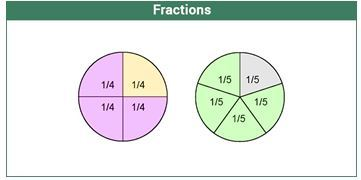### Representation of Fractions

A fraction is represented by 2 numbers on top of each other, separated by a line. The number on top is the numerator and the number below is the denominator. Example :34  which basically means 3 parts out of 4 equal divisions.

### Fractions on the Number Line

In order to represent a fraction on a number line, we divide the line segment between two whole numbers into n equal parts, where n is the denominator.
Example: To represent 15or35 , we divide the line between 0 and 1 in 5 equal parts. Then the numerator gives the number of divisions to mark.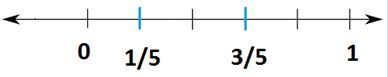## Multiplication of Fractions

### Multiplication of Fractions

Multiplication of a fraction by a whole number :
Example 1: 7×13=7×13=73
Example 2 : 5×745=3545, Dividing numerator and denominator by 5, we get79

Multiplication of a fraction by a fraction is basically product of numeratorsproduct of denominators
Example 1:  35×1213=3×125×13=3665
Example 2 : Multiplication of mixed fractions 423×117
First convert mixed fractions to improper fractions and then multiply
143×87=14×83×7=163

### Fraction as an Operator ‘Of’

The ‘of’ operator basically implies multiplication.

Example :16 of 18=16×18=186=3
or,12 of 11=12×11=112=512

## Division of Fractions

### Reciprocal of a Fraction

Reciprocal of any number n is written as1n
Reciprocal of a fraction is obtained by interchanging the numerator and denominator.
Example : Reciprocal of 25 is 52
Although zero divided by any number means zero itself, we cannot find reciprocals for them, as a number divided by 0 is undefined.
Example : Reciprocal of 0770

### Division of Fractions

Division of a whole number by a fraction : we multiply the whole number with the reciprocal of the fraction.
Example: 63÷75=63×57=9×5=45

Division of a fraction by a whole number: we multiply the fraction with the reciprocal of the whole number.
Example : 811÷4=811×14=211

Division of a fraction by another fraction : We multiply the dividend with the reciprocal of the divisor.
Example27÷521=27×215=65

## Types of Fractions

### Types of Fractions

Proper fractions represent a part of a whole. The numerator is smaller than the denominator.
Example: 14,79,5051. Proper fractions are greater than 0 and less than 1

Improper fractions have a numerator that is greater than or equal to the denominator.
Example : 456,65. Improper fractions are greater than 1 or equal to 1.

Mixed fractions are a combination of a whole number and a proper fraction.
Example : 435 can be written as 835.

Conversion of fractions : An improper fraction can be represented as mixed fraction and  a mixed fraction can represented as improper.
In the above case, if you multiply the denominator 5 with the whole number 8 add the numerator 3 to it, you get back 435

Like fractions : Fractions with the same denominator are called like fractions.
Example : 57,37. Here we can compare them as  57>37

Unlike fractions : Fractions with different denominators are called unlike fractions.
Example : 53,92. To compare them, we find the L.C.M of the denominator.
Here the L.C.M is 6 So, 5×23×2,9×32×3
106,276
276>106

## Decimals

### Introduction: Decimal

Decimal numbers are used to represent numbers that are smaller than the unit 1. Decimal number system is also known as base 10 system since each place value is denoted by a power of 10.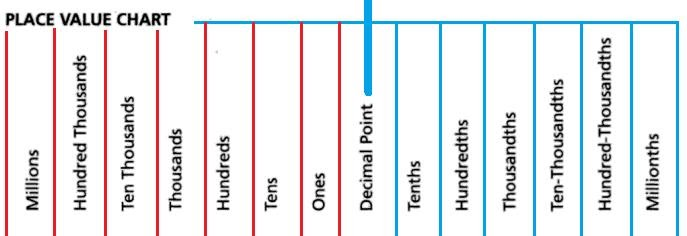A decimal number refers to a number consisting of the following two parts:
(i) Integral part (before the decimal point) (ii) Fractional Part (after the decimal point). These both are separated by a decimal separator(.) called the decimal point.
A decimal number is written as follows : Example 564.8 or 23.97.
The numbers to the left of the decimal point increase with the order of 10, while the numbers to the right of the point increase with the decrease order of 10.
The above example 564.8 can be read as ‘five hundred and sixty four and eight tenths’
5×100+6×10+4×1+8×110

A fraction can be written as a decimal and vice-versa. Example32=1.5 or 1.5=1510=32

### Multiplication of Decimals

Multiplication of decimal numbers with whole numbers :
Multiply them as whole numbers. The product will contain the same number of digits after the decimal point as that of the decimal number.
E.g : 11.3×4=45.2

Multiplication of decimals with powers of 10 :
If a decimal is multiplied by a power of 10, then the decimal point shifts to the right by the number of zeros in its power.
E.g : 45.678×10=456.78(decimal point shifts by 1 place to the right) or, 45.678×1000=45678 (decimal point shifts by 3 places to the right)

Multiplication of decimals with decimals :

Multiply the decimal numbers without decimal points and then give decimal point in the answer as many places same as the total number of places right to the decimal points in both numbers.

E.g :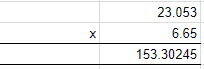### Division of Decimals

Dividing a decimal number by a whole number:
Example :  45.255
Step 1. Convert the Decimal number into Fraction: 45.25=4525100
Step 2. Divide the fraction by the whole number: 4525100÷5=(4525100)×(15)=9.05

Dividing a decimal number by a decimal number:
Example1 : 45.250.5
Step 1.Convert both the decimal numbers into fractions: 45.25=4525100 and 0.5=510
Step 2.Divide the fractions: 4525100÷510=4525100×105=90.5
Example 2: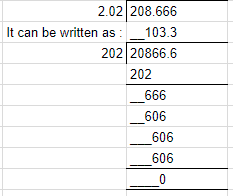Dividing a decimal number by powers of 10  :
If a decimal is divided by a power of 10, then the decimal point shifts to the left by the number of zeros present in the power of 10.
Example: 98.765÷100=0.98765Infinity

When the denominator in a fraction is very very small (almost tending to 0), then the value of the fraction tends towards infinity.
E.g : 9999990.000001=9999990000001 a very large number, which is considered to be